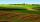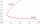Equations

Solve following system of equations:

6(x+7)+4(y-5)=12
2(x+y)-3(-2x+4y)=-44

Result

x =  -3
y =  2

Solution:

6(x+7)+4(y-5)=12
2(x+y)-3(-2x+4y)=-44

6x+4y = -10
8x-10y = -44

x = -3
y = 2

Calculated by our linear equations calculator.

Leave us a comment of example and its solution (i.e. if it is still somewhat unclear...):Be the first to comment!To solve this example are needed these knowledge from mathematics:

Do you have a system of equations and looking for calculator system of linear equations?

Next similar examples:

1. Equations - simpleSolve system of linear equations: x-2y=6 3x+2y=4
2. Linear systemSolve this linear system (two linear equations with two unknowns): x+y =36 19x+22y=720
3. TheatroTheatrical performance was attended by 480 spectators. Women were in the audience 40 more than men and children 60 less than half of adult spectators. How many men, women and children attended a theater performance?
4. Two numbersWe have two numbers. Their sum is 140. One-fifth of the first number is equal to half the second number. Determine those unknown numbers.
5. EurosPeter, Jane and Thomas have together € 550. Tomas has 20 euros more than Jane, Peter € 150 less than Thomas. Determine how much has each of them.
6. Waiting roomIn the waiting room are people and flies. Together they have 15 heads and 50 legs (fly has 6 legs). How many people and flies are in the waiting room?
7. Family parcelsIn father will he divided the land so that the older son had three bigger part than younger son. Later elder son gave 2.5 ha field to younger and they had both the same. Determine the area of family parcel.
8. SolutionsHow much 60% solution and how much 35% solution is needed to create 100 l of 40% solution?
9. Land areasTwo land areas is 244 m2. The first parcel is 40 m2 less than twice of the second one. What have acreage of each parcel?
10. TreesAlong the road were planted 250 trees of two types. Cherry for 60 CZK apiece and apple 50 CZK apiece. The entire plantation cost 12,800 CZK. How many was cherries and apples?
11. Three brothersThe three brothers have a total of 42 years. Jan is five years younger than Peter and Peter is 2 years younger than Michael. How many years has each of them?
12. MushroomsEva and Jane collected 114 mushrooms together. Eve found twice as much as Jane. How many mushrooms found each of them?
13. Hotel roomsIn the 45 rooms, there were 169 guests, some rooms were three-bedrooms and some five-bedrooms. How many rooms were?
14. SchoolsThree schools are attended by 678 pupils. To the first attend 21 students more and to the third 108 fewer students than to second school. How many students attend the schools?
15. The differenceThe difference of two numbers is 1375. If their exact quotient is 12. Find the two numbers
16. BlackberriesDaniel, Jolana and Stano collected together 34 blackberries. Daniel collected 8 blackberries more than Jolana, Jolana 4 more than Stano. Determine the number blackberries each collected .
17. SummerjobThree students participated in the summerjob. Altogether they earn 1780, -. Peter got a third less than John and Paul got 100,- more than Peter. How much got every one of them?